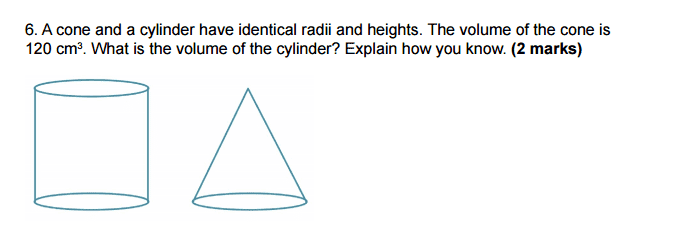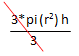# Volumes and heights of cones.

Kirito123

## Homework Statement## Homework Equations

volume of cone = pi(r)2h / 3
volume of cylinder = pi(r)2h

## The Attempt at a Solution

How can I do this, all they give is the volume and we know the value of pi ( 3.14159). I tried but you can't do it without knowing at least the radius or the height.

Kirito123
That is the exact wording there is nothing else to it, i might have not understood or it just doesn't make sense..

Dank2
That is the exact wording there is nothing else to it, i might have not understood or it just doesn't make sense..
By just looking at the equation you have written, What do you need to do the cylinder volume in order to get the cone volume ?

Dank2
you can't do it without knowing at least the radius or the height.
the height and radius is the same of both objects

Kirito123
yea they both have the same height and radius but i still can't find out the height or radius with just the volume.

Dank2
yea they both have the same height and radius but i still can't find out the height or radius with just the volume.
look again at the equations you have written, and only at them. what is the same and what is different, type it here.

Kirito123
volume of cone = pi(r)2h / 3
volume of cylinder = pi(r)2h

They both use pi and the radius is squared, only different is for a cone you have to divide by 3. I'm gonna take a crack at it but it might be wrong.
So to find the radius we would do as shown below :

120 x 3 (we are reversing the original formula)

360/ 3.14 = 114.64

114.64 squared are equal to 10.707.

Dank2
360/ 3.14 = 114.64
Why did you do that?

Kirito123
don't you multiply 3.14 by 360, the reverse of that would be division.

Dank2
don't you multiply 3.14 by 360, the reverse of that would be division.
no need to. we need to find the volume of the cylinder.

What happens if you multiply by 3 your first equation. don't use the 120 yet. just the first equation.

Kirito123
Sorry just so im clear what are you refering to when you say "first equations". Do you mean the volume of the cone or
120 x 3

Dank2
Sorry just so im clear what are you refering to when you say "first equations". Do you mean the volume of the cone or
this is the first equation you have written pi(r2)2h / 3 = Volume of cone

Dank2
Sorry just so im clear what are you refering to when you say "first equations". Do you mean the volume of the cone or
no numbers yet, just use the parameters, manually multiply it by 3 and type what you got

Kirito123
Ok just making sure so i don't mess up. If i multiply it by 3 it would become 3 Pi(h)r2??

Dank2
Ok just making sure so i don't mess up. If i multiply it by 3 it would become 3 Pi(h)r2??
wasn't there a /3 aswell? where did it go?

Kirito123
wait doesn't the multiplied 3 cancel out the other division one?

Dank2
wait doesn't the multiplied 3 cancel out the other division one?
Type it all down from the start, don't show only the result , and let's see

Kirito123
wait doesn't the multiplied 3 cancel out the other division one?

wait that only works if it was a division 3 that cancels a division 3.

Dank2
wait that only works if it was a division 3 that cancels a division 3.

1/3 * 3 = ? any division by three can be written as x/3 = x* (1/3)

Kirito123
it would = 1

Dank2
it would = 1
So looks like it would cancel one another. don't forget we need to multiply both sides of the equation always when we want to multiply one side we must multiply the other aswell, so equation will hold.

3* pi(r2)h / 3 = 3* Volume of cone = ?

Last edited:
Kirito123
sorry for late reply, anyway 3 * the volume of the cone would be 360.

Kirito123
since 3 x 120 = 360 as i said before right?

Dank2
since 3 x 120 = 360 as i said before right?

3* pi(r2)h / 3 = pi(r2)h = 3* Volume of cone
we multiplied by 3 so it cancels with /3, and the volume got multiplied by 3.

but what is pi(r2)h ? remember that r and h are the same

Kirito123
pi(r2)2h

Why did you put r2 and then 2h? and now i get what your saying, i have to find a radius and height that would be equal to 3 * volume of the cone?

Dank2
Why did you put r2 and then 2h? and now i get what your saying, i have to find a radius and height that would be equal to 3 * volume of the cone?

that was a mistake, fixed it.

3* pi(r2)h / 3 = pi(r2)h = 3* Volume of cone

take a look at your second equation that you have written at the first message. pi(r2)h is equal to what?

Dank2
Why did you put r2 and then 2h? and now i get what your saying, i have to find a radius and height that would be equal to 3 * volume of the cone?
Volume of the cylinder is equal to what?

Kirito123
its equal to 360 right. I'm saying this since if you where to multiply 3 x 120 you would get 360 and that 360 would represent pi(r2) h.

Dank2
Why did you put r2 and then 2h? and now i get what your saying, i have to find a radius and height that would be equal to 3 * volume of the cone?
it's written in the second equation, just need to copy paste really.

then plug it
its equal to 360 right. I'm saying this since if you where to multiply 3 x 120 you would get 360 and that 360 would represent pi(r2) h.
Summary:

Multiply first equation by 3 you get : 3* pi(r2)h / 3 = 3* Volume of cone. simplfy left side you get pi(r2)h = 3*Volume of cone.

but hey pi(r2)h is equal to volume of the cylinder from equation 2, so we plug it above, and get volume of the cylinder = 3*Volume of cone.

Now you do know the volume of the cone. and so we got the answer just by multiplying by 3.

Note that all of this holds because radius and height was the same.

Kirito123Pi(h)r2 ??

Kirito123
I was just reading over a lesson and i found this "Similar to the relationship between the prism and the pyramid, the volume of three cones fits into a cylinder with the same radius and height.", If im correct that's stating that 360 is the volume of the cylinder and 120 is the volume of the cone. I think that might be right ;3

In short 360 = volume of cylinder and 120 = volume of cones.

Gold Member
Write all equations and information to one equation system and think, if you can mathematically solve this for Vsilinder.